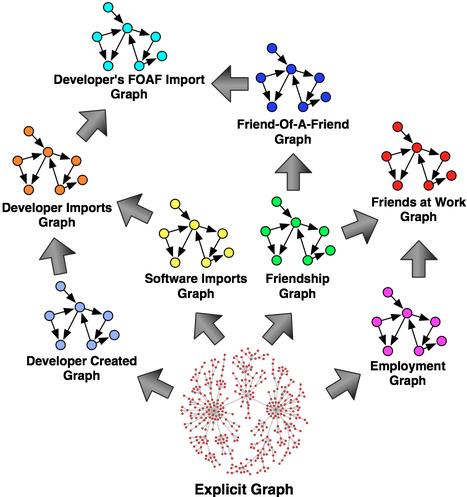# Learn About Property Graph Algorithms

· Database Zone · Interview
Save
6.89K Viewsthe term property graph has come to denote an attributed, multi-relational graph. that is, a graph where the edges are labeled and both vertices and edges can have any number of key/value properties associated with them. an example of a property graph with two vertices and one edge is diagrammed below.property graphs are more complex than the standard single-relational graphs of common knowledge. the reason for this is that there are different types of vertices (e.g. people , companies , software ) and different types of edges (e.g. knows , works_for , imports ). the complexities added by this data structure (and multi-relational graphs in general, e.g. rdf graphs) effect how graph algorithms are defined and evaluated.

standard graph theory textbooks typically present common algorithms such as various centralities , geodesics , assortative mixings , etc. these algorithms usually come pre-packaged with single-relational graph toolkits and frameworks (e.g. networkx , igraph ).

it is common for people to desire such graph algorithms when they begin to work with property graph software. i have been asked many times:

“does the property graph software you work on support any of the common centrality algorithms? for example, pagerank , closeness , betweenness , etc.?”

my answer to this question is always:

“what do you mean by centrality in a property graph?”

when a heterogeneous set of vertices can be related by a heterogeneous set of edges, there are numerous ways in which to calculate centrality ( or any other standard graph algorithm for that matter ).

1. ignore edge labels and use standard single-relational graph centrality algorithms.
2. isolate a particular “slice” of the graph (e.g. the knows subgraph) and use standard single-relational graph centrality algorithms.
3. make use of abstract adjacencies to compute centrality with higher-order semantics.

the purpose of this blog post is to stress point #3 and the power of property graph algorithms. in gremlin , you can calculate numerous eigenvector centralities for the same property graph instance. at this point, you might ask: “how can a graph have more than one primary eigenvector?” the answer lies in seeing all the graphs that exist within the graph—i.e. seeing all the higher-order, derived, implicit, virtual, abstract adjacencies. each line below exemplifies point #1, #2, and #3 in the list above, respectively. the code examples use the power method to calculate the vertex centrality rankings which are stored in the map m .

```g.v.oute.inv.groupcount(m).loop(3){c++ < 10000} // point #1
g.v.oute[[label:'knows']].inv.groupcount(m).loop(4){c++ < 10000} // point #2
g.v.???.groupcount(m).loop(?){c++ < 10000} // point #3
```

the ??? on line 3 refers to the fact that ??? can be any arbitrary computation. for example, ??? can be:

```oute[[label:'works_for']].inv.ine[[label:'works_for']].outv
oute[[label:'works_for']].inv[[name:'acme']].ine[[label:'works_for']].outv
oute[[label:'develops']].inv.oute[[label:'imports']].inv[[name:'blueprints']].back(7).oute[[label:'works_for']].inv.ine[[label:'works_for']].outv.oute[[label:'develops']].inv.oute[[label:'imports']].inv[[name:'blueprints']].back(7)
```these ideas are explored in more detail in the following article and slideshow.

rodriguez m.a., shinavier, j., “ exposing multi-relational networks to single-relational network analysis algorithms ,” journal of informetrics, 4(1), pp. 29-41, elsevier, doi:10.1016/j.joi.2009.06.004, 2009.

Topics:

Opinions expressed by DZone contributors are their own.